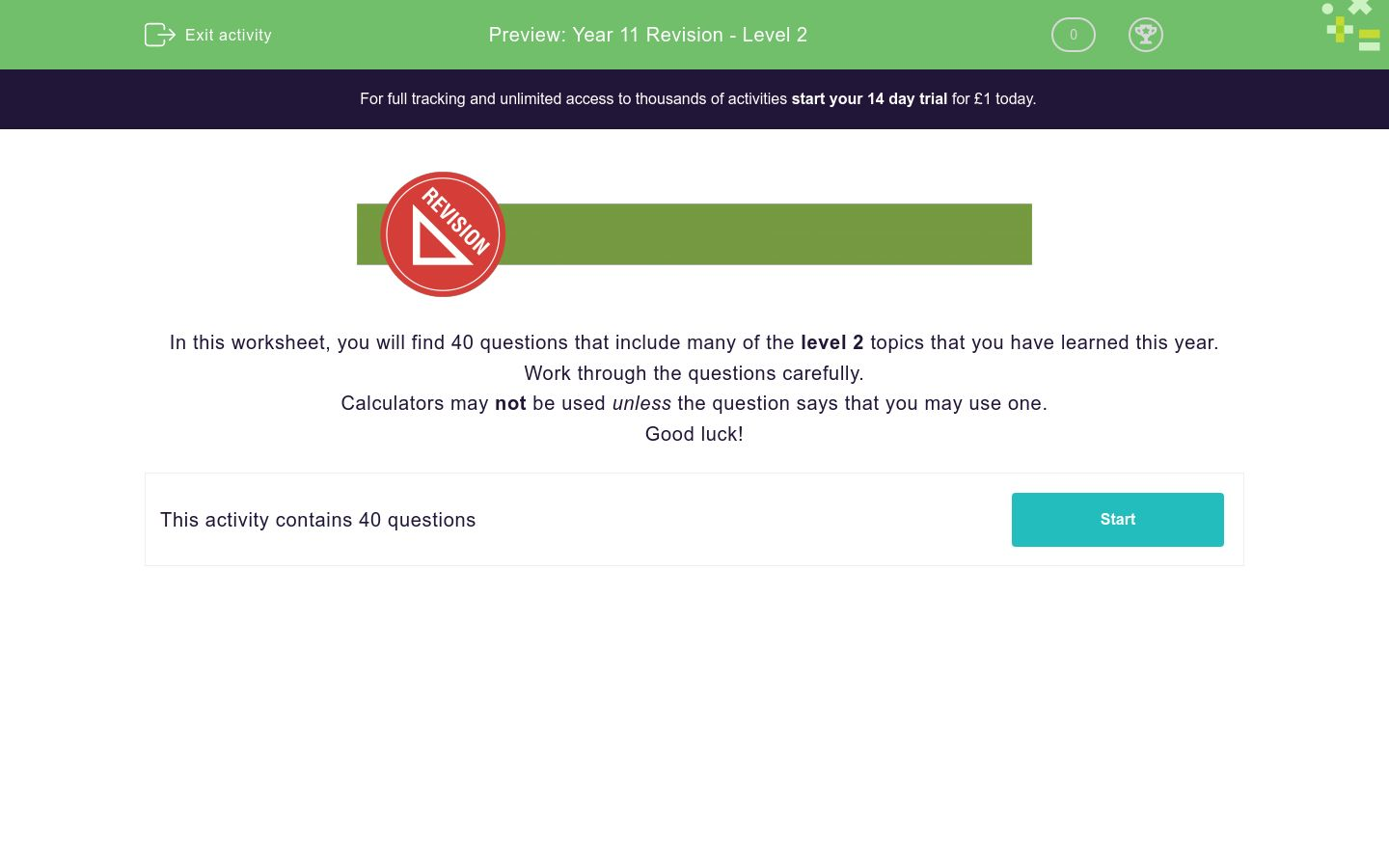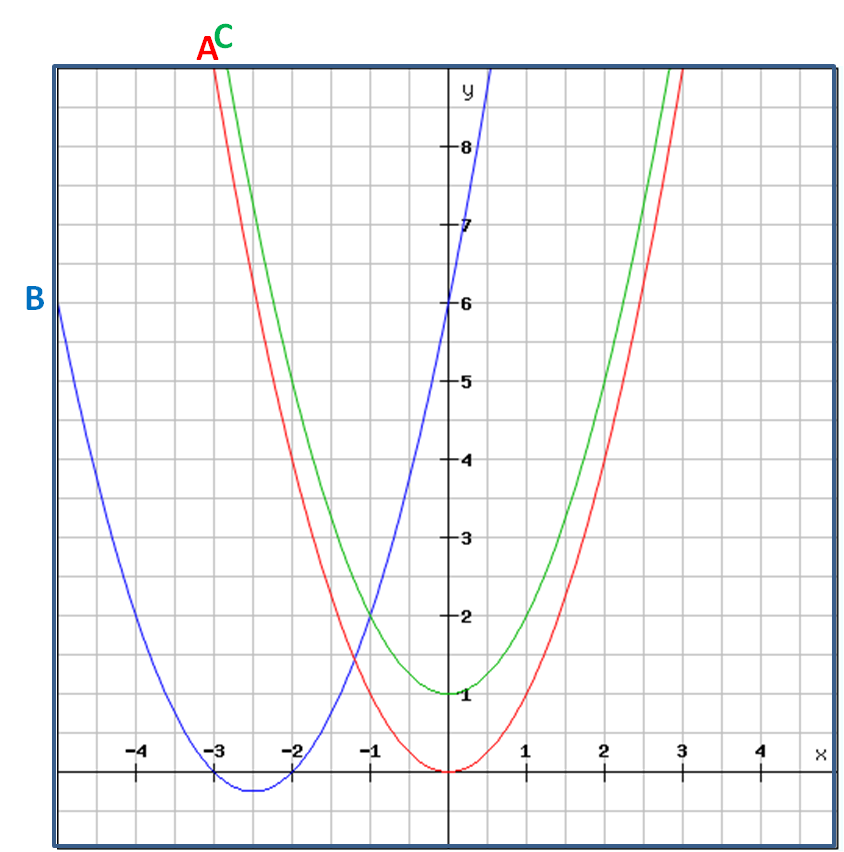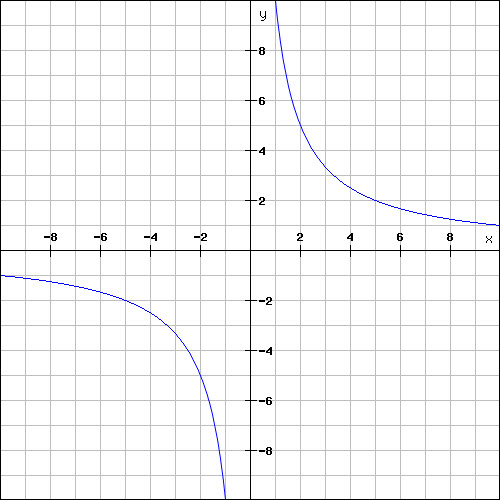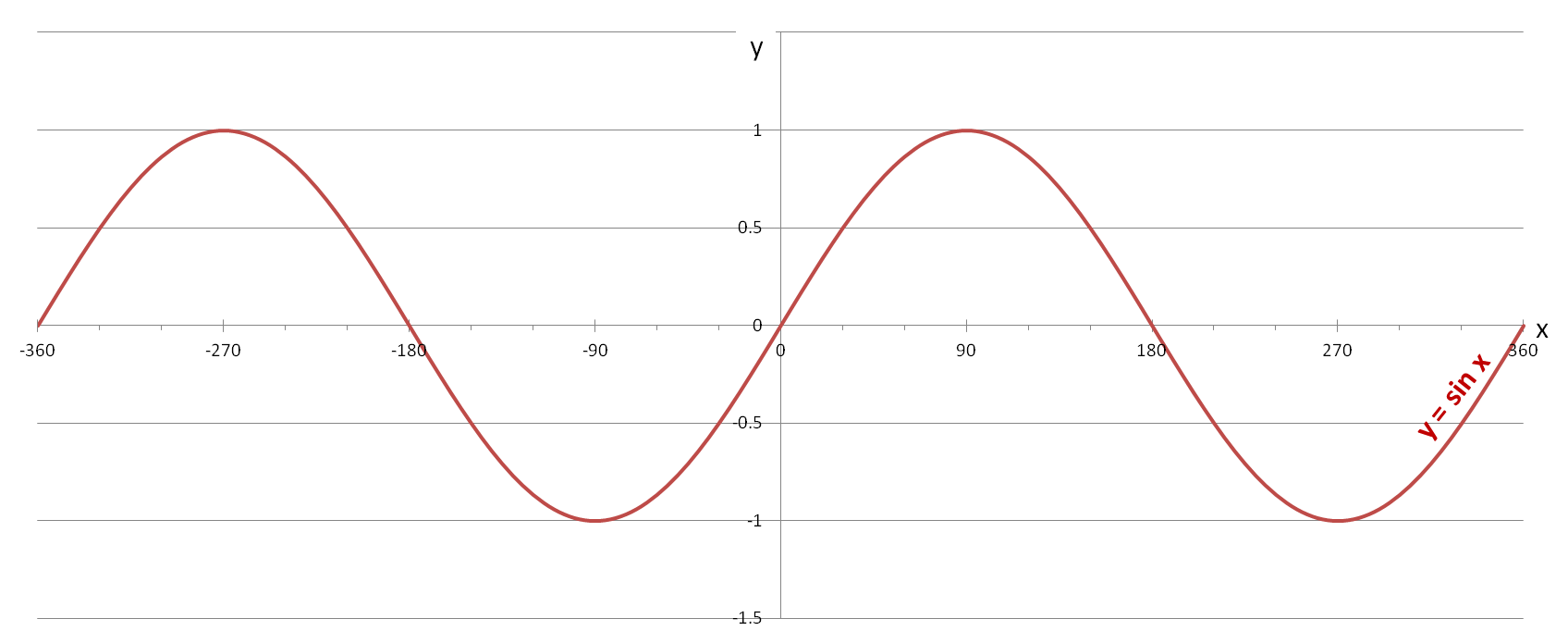# Year 11 Revision - Level 2

This is a level 2 revision exercise to help prepare for Year 11/end of Key Stage 4 tests.Key stage:  KS 4

Curriculum topic:  Revision

Curriculum subtopic:  Year 11 Revision

Difficulty level:### QUESTION 1 of 10In this worksheet, you will find 40 questions that include many of the level 2 topics that you have learned this year.

Work through the questions carefully.

Calculators may not be used unless the question says that you may use one.

Good luck!

Is the following number rational or irrational?

3√729

rational

irrationalYou may use a calculator for this question.

Select the recurring decimal which is equal to:

 127 2251.

2.

3.

Work out, without a calculator:

91/3 × 31/3

Work out:

 7 - 5 × 7 15 6 10

(Give your answer as a fraction, reduced to its lowest terms, e.g. 7/10.)

Place these numbers in ascending order of size, by converting them to decimals first:

8.9%     1/11     9 × 10-2     0.01½     0.312

## Column B

1 (smallest)
1/11
2
8.9%
3
9 × 10-2
4
0.312
5 (largest)
0.01½

The number n has been rounded to the nearest thousand, to get 73 000.

A and B are the upper and lower bounds for n, such that  A ≤ n < B.

Select the correct values for A and B, the lower and upper bounds.

(Write the number without spaces between the numbers.)

 value A B

Rationalise the denominator of this fraction giving your answer as a surd in the form a√b, where a and b are integers and b is as small as possible.

 2 √3
5√25

(√5)/5

(2√3)/3

Subtract:

3 × 102  -   4 × 10-1

3.4 × 101

2.996 × 102

2.6 × 102

Rearrange the following equation so that it is in the form ax + by = c,

where a ≥ 0, a, b and c are integers and the HCF of a, b, and c is 1.

½y = -4 - 2x

4x + y = 8

4x + y = -8

4x + 2y = -8

Solve the following equation for x:

 x - 4x = -6 2 5

(Just write the number.)

4x2 + 39x - 10

(4x - 5) (x + 2)

(4x + 1) (x - 10)

(x - 2) (4x + 5)

(4x - 1) (x + 10)

3x2 - 19x + 2 = 0

x = -3.23

x = 6.23

x = -4.107

x = 0.107

Select all the possible equations for the curve labelled B below.y = x² - 5x - 6

y = (x + 2) (x + 3)

y = (x - 2) (x - 3)

y = (x + 3) (x + 2)

y = x² + 5x + 6

Find the y-intercept of the graph with the following equation:

(i.e. where the line crosses the y-axis)

3x - 12y = 6

(0, -2)

(2, 0)

(-½, 0)

(0, -½)

Divide the following expression by  2x.

4x4 - 6x3 + 10x2 - 2x

8x6 - 2x3 + 5x2 - x

2x4 - 3x3 + 5x2 - x

2x3 - 3x2 + 5x - 1

Find the gradient of a line perpendicular to the line with equation:

5x - 10y = 2

Solve for x:

6x3 - 109 = -365 + 2x3

(Just write the number.)

Simplify the following algebraic fraction by first factorising the top or the bottom.

 49x8 - 35x5 7x5 - 5x2
7x4

7x3

7x2You may use a calculator for this question.

Bill invests £1000 at 2.5% interest p.a.

How much compound interest is earned in pounds after three years?

(Just write the number with 2 decimal places.)You may use a calculator for this question.

Given that y is directly proportional to x and that x = 19 when y = 123.5,

find the value of x when y = 29.25.

3 mice take 8 days to eat a block of cheese.

How many days would it take 4 mice to eat a similar block of cheese?

(Just write the number.)You may use a calculator for this question.

A bank pays 4% compound interest.

If I invest £100 now, how many years will it take for me to double my money at this fixed rate?

(Just write the number.)You may use a calculator for this question.

Look at the function:

f(t) = 3t + 10

Work out f(7) - f(5).

Look at this graph.

What is the equation of the blue line?y = 10x

y = 10/x

y = 10x²

y = 10x³You may use a calculator for this question.Calculate the curved surface area (in cm2) of a cone with radius R equal to 3 cm and height H equal to 4 cm.You may use a calculator for this question.

Calculate the length of the following vector to 3 sig. figs.:

 ( -8 ) -9You may use a calculator for this question.

Find the length of the cuboid's diagonal AG of this 4 cm × 5 cm × 10 cm cuboid in cm to 3 sig. figs.

(Just write the number.)Without using a calculator, state the value of:

cos 30°

2/√3

√3/2

√2/3

3/2

3/√2

Use the sine graph shown below to state the value of:

sin 330°You may use a calculator for this question.

Fred's eye level is 1.6 metres above ground.

He is standing on a cliff which is h metres above sea level and measures the angle of depression to a boat 400 m away as 26°.

Calculate the height of the cliff, h, in metres.You may use a calculator for this question.

In triangle PQR, which is not drawn to scale,

PR = 19 cm, ∠PQR = 102° and ∠PRQ = 37°.

Use the sine rule to find the length of PQ in cm to 1 dp.

(Just write the number.)You may use a calculator for this question.

In triangle PQR, which is not drawn to scale,

PQ = 4.5 cm, QR = 10 cm, and PR = 12 cm.

Use the cosine rule to find ∠PRQ to the nearest degree.

(Just write the number.)A money bag contains 20 × £1 coins, 25 × 50p coins, n × 20p coins, 10 × 10p coins and 5 × 2p coins.

The probability that a 10p coin is selected at random is 2/19.

Calculate n, the number of 20p coins in the bag.

Two fair dice are thrown.

What is the probability that the two dice will both show prime numbers?

(Write your answer as a fraction in the form a/b in its lowest terms.)

Mr. Brown chose the children who came in the top ten positions in an English exam to survey them about a new novel.

Is the sample likely to be biased?

yes

no

A shopping centre has 3 entrances, A, B and C.

They are being repaired and only one entrance will ever be open at any one time.

The probability that Entrance A is open is 2/5. The probability that Entrance B is open is 1/4.

What is the probability that Entrance C is open?

(Write your answer as a fraction in the form a/b in its lowest terms.)

A survey was carried out to record the marks some students scored in a test.

The marks were as follows:

25, 51, 20, 25, 18, 40, 30, 30, 40, 30, 19

Which box plot shows this information?A

B

C

none of them

A survey of 100 people was carried out to see how many times they checked their email at the weekend.

The frequencies and cumulative frequencies are shown in the table below.

Calculate the value of C in this table.

Times (n) freq (f) cumulative freq
0 ≤ n ≤ 5 A 7
5 < n ≤ 10 9
10 < n ≤ 15 B 55
15 < n ≤ 20 35
20 < n ≤ 25 C
Total  100

Find the lower quartile of the following set of data:

16     9     1    15     11    14        10    4    3

The ages of 100 young people attending a holiday club are recorded.

The following table shows the distribution of ages.

Age (years) frequency (f)
0 < a < 5 5
5 ≤ a < 10 15
10 ≤ a < 16 24
16 ≤ a < 18 38
18 ≤ a < 30 18
Total 100

Work out the Frequency Density for the 18 ≤ a < 30 class interval.

• Question 1

Is the following number rational or irrational?

3√729

rational
EDDIE SAYS
³√729 = 9 which can be written as 9/1.
• Question 2You may use a calculator for this question.

Select the recurring decimal which is equal to:

 127 2252.
EDDIE SAYS
127 ÷ 225 = 0.5644444444...
The 4 recurs.
A dot only goes on the 4 but not on the 5 or 6, because these digits don't recur.
• Question 3

Work out, without a calculator:

91/3 × 31/3

3
EDDIE SAYS
= (9 × 3)1/3
= 271/3
= 3√27
= 3
• Question 4

Work out:

 7 - 5 × 7 15 6 10

(Give your answer as a fraction, reduced to its lowest terms, e.g. 7/10.)

-7/60
EDDIE SAYS
First work out 5/6 × 7/10 = 7/12.
Then work out 7/15 - 7/12 = 28/60 - 35/60 = -7/60.
• Question 5

Place these numbers in ascending order of size, by converting them to decimals first:

8.9%     1/11     9 × 10-2     0.01½     0.312

## Column B

1 (smallest)
8.9%
2
9 × 10-2
3
1/11
4
0.312
5 (largest)
0.01½
EDDIE SAYS
8.9% = 0.089
9 × 10-2 = 0.09
1/11 = 0.0909...
0.312 = 0.0961
0.01½ = √0.01 = 0.1
• Question 6

The number n has been rounded to the nearest thousand, to get 73 000.

A and B are the upper and lower bounds for n, such that  A ≤ n < B.

Select the correct values for A and B, the lower and upper bounds.

(Write the number without spaces between the numbers.)

 value A B
EDDIE SAYS
The number n lies between 72 500 and 73 500, in order for it to be rounded to 73 000 to the nearest thousand.
• Question 7

Rationalise the denominator of this fraction giving your answer as a surd in the form a√b, where a and b are integers and b is as small as possible.

 2 √3
(2√3)/3
EDDIE SAYS
Multiply top and bottom by √3.
• Question 8

Subtract:

3 × 102  -   4 × 10-1

2.996 × 102
EDDIE SAYS
3 × 102 = 300
4 × 10-1 = 0.4
300 - 0.4 = 299.6 = 2.996 × 102
• Question 9

Rearrange the following equation so that it is in the form ax + by = c,

where a ≥ 0, a, b and c are integers and the HCF of a, b, and c is 1.

½y = -4 - 2x

4x + y = -8
EDDIE SAYS
½y = -4 - 2x
2x + ½y = -4
4x + y = -8
• Question 10

Solve the following equation for x:

 x - 4x = -6 2 5

(Just write the number.)

20
EDDIE SAYS
Multiply each term by 10 to get:
5x - 8x = -60
-3x = -60
x = 20
• Question 11

4x2 + 39x - 10

(4x - 1) (x + 10)
EDDIE SAYS
We look for factor pairs that multiply to give -40 and add to give +39.
These are 40 and -1.
Factorise 4x² + 40x - x - 10 in pairs.
• Question 12

3x2 - 19x + 2 = 0

x = 6.23
x = 0.107
EDDIE SAYSa=3 b=-19 c=2
• Question 13

Select all the possible equations for the curve labelled B below.y = (x + 2) (x + 3)
y = (x + 3) (x + 2)
y = x² + 5x + 6
EDDIE SAYS
The line passes through (-3, 0) and (-2, 0), so y = (x + 2)(x + 3).
This is y = x² + 5x + 6.
• Question 14

Find the y-intercept of the graph with the following equation:

(i.e. where the line crosses the y-axis)

3x - 12y = 6

(0, -½)
EDDIE SAYS
When x = 0,
-12y = 6
y = -½
• Question 15

Divide the following expression by  2x.

4x4 - 6x3 + 10x2 - 2x

2x3 - 3x2 + 5x - 1
EDDIE SAYS
 4x4 - 6x3 + 10x2 - 2x 2x 2x 2x 2x
• Question 16

Find the gradient of a line perpendicular to the line with equation:

5x - 10y = 2

-2
EDDIE SAYS
5x - 2 = 10y
y = ½x - 0.2 has a gradient of ½.
Product of gradients of perpendicular lines is always -1, so gradient of perpendicular line will be -2, since -2 × ½ = -1.
• Question 17

Solve for x:

6x3 - 109 = -365 + 2x3

(Just write the number.)

-4
EDDIE SAYS
6x³ - 109 = -365 + 2x³
Add 109 and subtract 2x³ from both sides to get:
4x³ = -256
Divide both sides by 3 to get:
x³ = -64
Cube root both sides to get:
x = ³√-64 = -4
• Question 18

Simplify the following algebraic fraction by first factorising the top or the bottom.

 49x8 - 35x5 7x5 - 5x2
7x3
EDDIE SAYS
 7x3(7x5 - 5x2) 7x5 - 5x2
• Question 19You may use a calculator for this question.

Bill invests £1000 at 2.5% interest p.a.

How much compound interest is earned in pounds after three years?

(Just write the number with 2 decimal places.)

76.89
EDDIE SAYS
1000 × 1.025³ - 1000
• Question 20You may use a calculator for this question.

Given that y is directly proportional to x and that x = 19 when y = 123.5,

find the value of x when y = 29.25.

4.5
EDDIE SAYS
y = kx
123.5 = 19k
k = 123.5 ÷ 19 = 6.5
y = 6.5x
x = y ÷ 6.5 = 29.25 ÷ 6.5 = 4.5
• Question 21

3 mice take 8 days to eat a block of cheese.

How many days would it take 4 mice to eat a similar block of cheese?

(Just write the number.)

6
EDDIE SAYS
It would take 1 mouse 24 days.
• Question 22You may use a calculator for this question.

A bank pays 4% compound interest.

If I invest £100 now, how many years will it take for me to double my money at this fixed rate?

(Just write the number.)

18
EDDIE SAYS
1.0417 = 1.9479...
1.0418 = 2.0258...
• Question 23You may use a calculator for this question.

Look at the function:

f(t) = 3t + 10

Work out f(7) - f(5).

1944
EDDIE SAYS
35 + 10 = 253
37 + 10 = 2197
Difference = 2197 - 253 = 1944
• Question 24

Look at this graph.

What is the equation of the blue line?y = 10/x
EDDIE SAYS
Test out coordinates, e.g. when x = 2, y = 5, and when x = 5, y = 2.
This is the exponential curve y = 10/x.
• Question 25You may use a calculator for this question.Calculate the curved surface area (in cm2) of a cone with radius R equal to 3 cm and height H equal to 4 cm.

47.1
EDDIE SAYS
This is a 3, 4, 5 Pythagorean Triad, so slant length, l, = 5.
Curved Surface Area = π × R × l = π × 3 × 5
• Question 26You may use a calculator for this question.

Calculate the length of the following vector to 3 sig. figs.:

 ( -8 ) -9

12.0
EDDIE SAYS
a² = (-8)² + (-9)² = 64 + 81 = 145
• Question 27You may use a calculator for this question.

Find the length of the cuboid's diagonal AG of this 4 cm × 5 cm × 10 cm cuboid in cm to 3 sig. figs.

(Just write the number.)11.9
EDDIE SAYS
Use Pythagoras' Theorem to get:
AG² = 4² + 5² + 10² = 16 + 25 + 100 = 141
• Question 28

Without using a calculator, state the value of:

cos 30°

√3/2
EDDIE SAYSIn the blue triangle, cos 30° = adj/hyp = √3/2
• Question 29

Use the sine graph shown below to state the value of:

sin 330°-0.5
EDDIE SAYS
sin330° = - sin30° = -0.5
• Question 30You may use a calculator for this question.

Fred's eye level is 1.6 metres above ground.

He is standing on a cliff which is h metres above sea level and measures the angle of depression to a boat 400 m away as 26°.

Calculate the height of the cliff, h, in metres.193.5
EDDIE SAYS
(h + 1.6)/400 = tan26°
h + 1.6 = 400tan26° = 195.093035
h = 193.493...
• Question 31You may use a calculator for this question.

In triangle PQR, which is not drawn to scale,

PR = 19 cm, ∠PQR = 102° and ∠PRQ = 37°.

Use the sine rule to find the length of PQ in cm to 1 dp.

(Just write the number.)11.7
EDDIE SAYS
 PQ = 19 sin 37° sin 102°
• Question 32You may use a calculator for this question.

In triangle PQR, which is not drawn to scale,

PQ = 4.5 cm, QR = 10 cm, and PR = 12 cm.

Use the cosine rule to find ∠PRQ to the nearest degree.

(Just write the number.)21
EDDIE SAYS
4.5² = 10² + 12² - 2 × 10 × 12 × cosθ°
cosθ° = (4.5² - 10² - 12²) ÷ -(2 × 10 × 12) = 0.93229...
θ° = 21.205...
• Question 33

A money bag contains 20 × £1 coins, 25 × 50p coins, n × 20p coins, 10 × 10p coins and 5 × 2p coins.

The probability that a 10p coin is selected at random is 2/19.

Calculate n, the number of 20p coins in the bag.

35
EDDIE SAYS
10/(60 + n) = 2/19
190 = 2(60 + n)
190 = 120 + 2n
2n = 70
n = 35
• Question 34

Two fair dice are thrown.

What is the probability that the two dice will both show prime numbers?

(Write your answer as a fraction in the form a/b in its lowest terms.)

1/4
EDDIE SAYSThere are 9 results that have double prime numbers out of 36.
(2, 2) (2, 3) (2, 5)
(3, 2) (3, 3) (3, 5)
(5, 2) (5, 3) (5, 5)
• Question 35

Mr. Brown chose the children who came in the top ten positions in an English exam to survey them about a new novel.

Is the sample likely to be biased?

yes
EDDIE SAYS
Those who were not in the top ten may have completely different opinions.
• Question 36

A shopping centre has 3 entrances, A, B and C.

They are being repaired and only one entrance will ever be open at any one time.

The probability that Entrance A is open is 2/5. The probability that Entrance B is open is 1/4.

What is the probability that Entrance C is open?

(Write your answer as a fraction in the form a/b in its lowest terms.)

7/20
EDDIE SAYS
1 - 1/4 - 2/5 = 20/20 - 5/20 - 8/20 = 7/20
• Question 37

A survey was carried out to record the marks some students scored in a test.

The marks were as follows:

25, 51, 20, 25, 18, 40, 30, 30, 40, 30, 19

Which box plot shows this information?B
EDDIE SAYS
First place the marks in ascending order.
Minimum mark = 18
Lower quartile = 20
Median = 30
Upper quartile = 40
Maximum mark = 51
• Question 38

A survey of 100 people was carried out to see how many times they checked their email at the weekend.

The frequencies and cumulative frequencies are shown in the table below.

Calculate the value of C in this table.

Times (n) freq (f) cumulative freq
0 ≤ n ≤ 5 A 7
5 < n ≤ 10 9
10 < n ≤ 15 B 55
15 < n ≤ 20 35
20 < n ≤ 25 C
Total  100

10
EDDIE SAYS
A = 7
B = 55 - 9 - 7 = 39
C = 100 - 35 - 39 - 9 - 7 = 10
• Question 39

Find the lower quartile of the following set of data:

16     9     1    15     11    14        10    4    3

3.5
EDDIE SAYS
Put the numbers in order first to get 1, 3, 4, 9, 10, 11, 14, 15, 16.
Median of 1, 3, 4, 9 is 3.5.
• Question 40

The ages of 100 young people attending a holiday club are recorded.

The following table shows the distribution of ages.

Age (years) frequency (f)
0 < a < 5 5
5 ≤ a < 10 15
10 ≤ a < 16 24
16 ≤ a < 18 38
18 ≤ a < 30 18
Total 100

Work out the Frequency Density for the 18 ≤ a < 30 class interval.

1.5
EDDIE SAYS
Class width = 30 - 18 = 12
Frequency Density = 18 ÷ 12 = 1.5
---- OR ----

Sign up for a £1 trial so you can track and measure your child's progress on this activity.

### What is EdPlace?

We're your National Curriculum aligned online education content provider helping each child succeed in English, maths and science from year 1 to GCSE. With an EdPlace account you’ll be able to track and measure progress, helping each child achieve their best. We build confidence and attainment by personalising each child’s learning at a level that suits them.